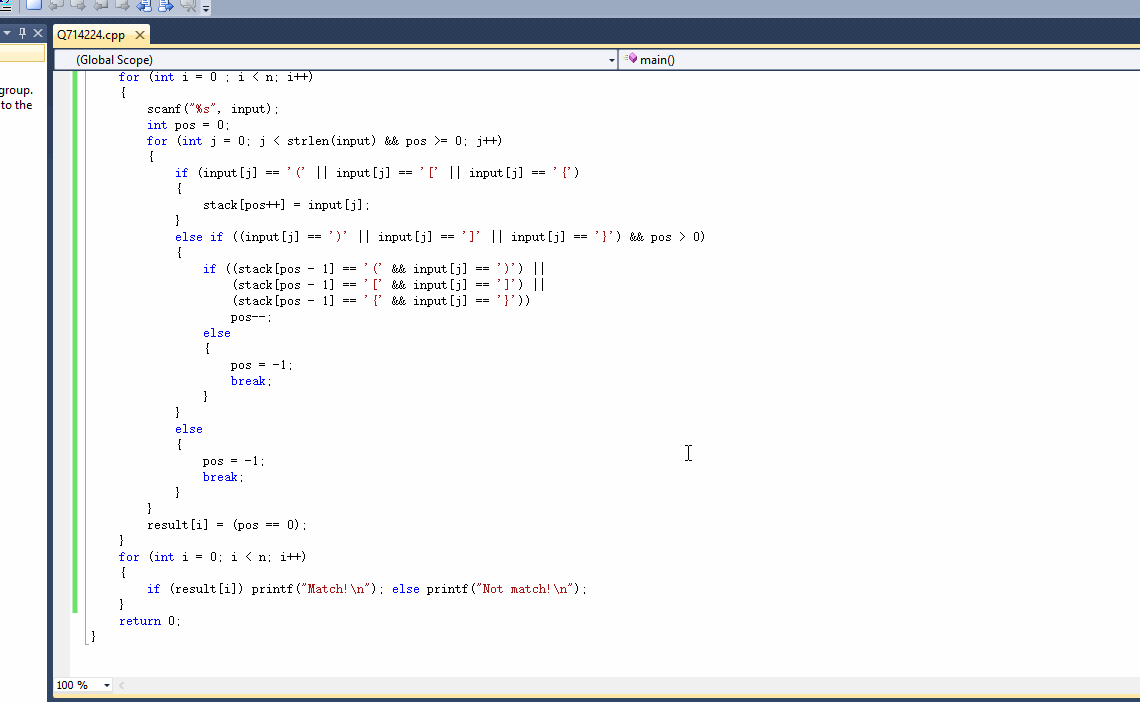C语言写一算法，对输入的表达式中括号匹配情况检查。匹配的括号需要成对出现，且不嵌套。

4
([{}()])
([({}))
[(})({})
([{})]

Match!
Not match!
Not match!
Not match!

1个回答

``````// Q714224.cpp : Defines the entry point for the console application.
//

#include "stdafx.h"

#include "stdio.h"
#include "stdlib.h"
#include "string.h"

int main()
{
int n;
char input;
char stack;
scanf("%d", &n);
int *result = (int *)malloc(sizeof(int) * n);
for (int i = 0 ; i < n; i++)
{
scanf("%s", input);
int pos = 0;
for (int j = 0; j < strlen(input) && pos >= 0; j++)
{
if (input[j] == '(' || input[j] == '[' || input[j] == '{')
{
stack[pos++] = input[j];
}
else if ((input[j] == ')' || input[j] == ']' || input[j] == '}') && pos > 0)
{
if ((stack[pos - 1] == '(' && input[j] == ')') ||
(stack[pos - 1] == '[' && input[j] == ']') ||
(stack[pos - 1] == '{' && input[j] == '}'))
pos--;
else
{
pos = -1;
break;
}
}
else
{
pos = -1;
break;
}
}
result[i] = (pos == 0);
}
for (int i = 0; i < n; i++)
{
if (result[i]) printf("Match!\n"); else printf("Not match!\n");
}
return 0;
}

```````如果问题得到解决，请点我回答左上角的采纳和向上的箭头，谢谢`-

-